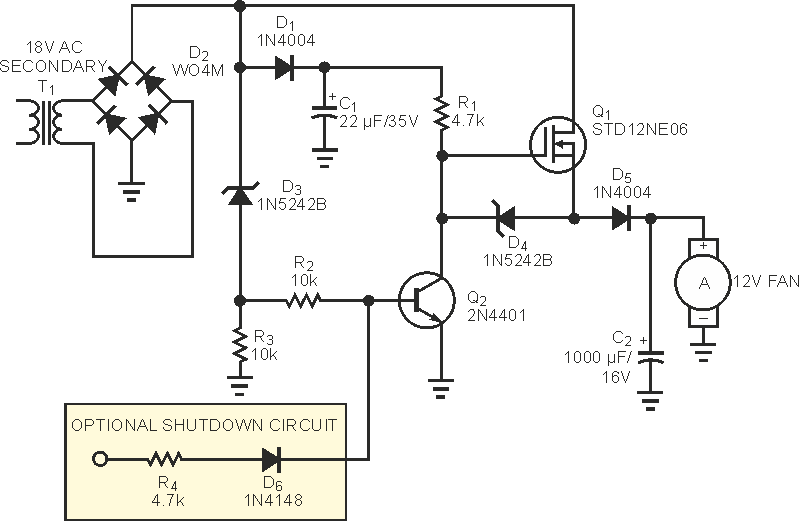# MOSFET switch provides efficient ac/dc conversion

## STMicroelectronics STD12NE06

Spehro Pefhany

EDN

Occasionally, you have access to a transformer for powering a dc circuit, but its output voltage is much higher than that required for the dc voltage. The full-wave-rectified and filtered output of an ac input voltage VX, is

VDC = 1.414VX – 2VF,

where VF is the forward drop in the rectifier (approximately 0.7 V). For example, if you require 12 V dc to power a small cooling fan drawing 100 mA and the ac voltage is 18 V, a full-wave rectifier and filter results in a 24 V-dc output. Although you can regulate the voltage down to 12 V dc by using a simple three-terminal regulator (such a µA7812), the result is wasted power of approximately 1.3 W. This waste means that you must provide for heat removal, somewhat defeating the purpose of including the cooling fan. If you use a typical 100 × 100-mm, 12 V-dc fan rated at 0.45 A, the typical heat loss is approximately 2.5 W, increasing to 5 W at full load. In many applications, this level of loss is unacceptable, so you'd have to use an extra transformer secondary, a dc/dc converter, or a switching regulator.

The circuit in Figure 1 uses a MOSFET switch and diode to effectively draw current from the transformer when the voltage is close to the desired level of 12 V dc.Figure 1. Using a MOSFET circuit, you can efficiently convert the too-high voltage of a leftover transformer to a lower dc level.

The full-wave bridge, D2, rectifies the 18 V-ac signal. The diode, D1, and C1 provide a gate bias voltage of approximately 24 V dc. This voltage drives the gate of Q1 through R1, shunted by D4, which maintains the gate voltage at a maximum of 12 V relative to the source, even during transient conditions. As the bridge-rectifier output increases from 0 V to the peak of approximately 24 V each half-cycle, the bias voltage holds the MOSFET on until the input voltage reaches the breakdown voltage of D3 (12 V) plus the VBE(ON) of Q2, or approximately 12.7 V. At that point, Q2 turns on, turning Q1 off. The output filter capacitor, C2, charges through D5. As the rectifier output voltage decreases from 24 to 0 V, Q2 again turns off at approximately 12.7 V, allowing Q1 to turn on and provide another pulse of current to charge C2. C2 provides power for the load between the pulses, which occur at 240 Hz with a 60-Hz input. Thus, power drain from the transformer occurs in short pulses, much in the manner of a typical bridge-rectifier/output-filter arrangement but at double the frequency. If you want to turn the fan off with a logic signal, you can add R4 and D6. When you apply a logic-high signal to the input, Q2 conducts, turning the MOSFET off.

EDN